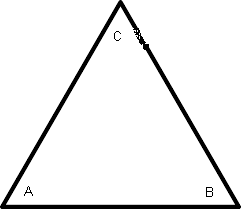# Triangle Calculator

Calculate the various properties of triangle for given values.Area of triangle: [ l×b/2 ] Enter the length and breadth = Area of a triangle =Perimeter of Triangle:[ (a + b + c) ] Enter the length and breadth = Perimeter of a triangle =Area of Equilateral Triangle:[ (Sqrt(3)/4)×(side)² ] Enter the length = Area of a equilateral triangle=Area of Triangle SAS(2sides & opposite angle):[ ½×a×b×SinC ] Enter the length and breadth = Enter Angle = Area of a triangle SAS=

Type of triangles with definition
 Triangle Type Definition Equiangular triangle The triangle which has all three interior angles equal having every angle equals to 60°, because always the sum of all three interior angles are 180°. The equiangular triangle has all three sides in same length (congruent). Equilateral triangle The triangle which has all three sides equal in length and also having every interior angle equals to 60°, because always the sum of all three interior angles are 180°. Right triangles The triangle which has one right angle (90 degrees) out of its interior angles. Obtuse triangle The triangle which has one angle > 90 degrees out of its interior angles. Acute triangle The triangle which has all the interior angles < 90 degrees. Scalene triangles The triangle where every side has different length. The randomly drawn triangles having all three different interior-angles called scalene triangle. The triangle is scalene if all the sides has different lengths or all three angles are different. Isosceles triangles The triangle where two sides has same length. The isosceles triangle also has two interior-angles of same degree. In the case of isosceles triangle we can find the other two interior angle if one interior angle is given.